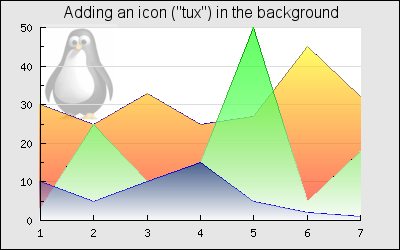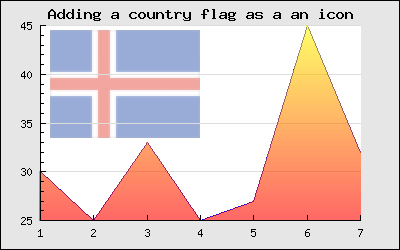## 14.14. Adding icons (and small images) to the graph

In addition to the standard background image you can also add an arbitrary number of icons onto the background of the graph. These icons are created with a call to the special Plot `class IconPlot` defined in the module "`jpgraph_iconplot.php`". The image which is the base of the icons can be

1. an arbitrary image from a file

2. one of the built-in country flags

The basic structure of adding an icon somewhere on the graph is to first create an instance of the IconPlot class and then position the icon at the wanted x,y-position on the graph and finally add the object to the graph using the standard `Graph::Add()` method. The constructor for the IconPlot class have the following signature

• `__construct(\$aFile='',\$aX=0,\$aY=0,\$aScale=1.0,\$aMix=100)`

The parameters should be self explanatory. The `\$aMix` factor specifies the degree of alpha blending between the background and the icon. A value of 100 means no blending and a value of 0 means that the icon is not shown at all, only the background.

Some useful methods in this class are

• `SetCountryFlag(\$aFlag,\$aX=0,\$aY=0,\$aScale=1.0,\$aMix=100,\$aStdSize=3)`

This method specifies that the specified country flag should be used

• `SetPos(\$aX,\$aY)`

Same as the optional arguments in th constructor. The coordinates are specified as absolute pixels where (0,0) is the top left corner.

• `CreateFromString(\$aStr)`

Creates the image from a string instead of reading it from a file.

• `SetScalePos(\$aX,\$aY)`

Specifes the potision using the scale coordinate as specified by the x- and y-scales

• `SetAnchor(\$aXAnchor='left',\$aYAnchor='center')`

Sets the anchor point of the icon, i.e. the position in the icon that should be aligned with the specified icon position in the graph.

The following short example shows how an icon is created and added to a graph

 ```1 2 3 ``` ```\$icon = new IconPlot('myimage.png',0.2,0.3,1,30); \$icon->SetAnchor('center','center'); \$graph->Add(\$icon);```

It is possible to control how much of the icon should be blended into the background by specifying a percentage (1-100). The example below in Figure 14.84 shows how to mix in the picture of "Tux the penguin" into the background of a filled line graph.

Figure 14.84. Mixing an icon image into the background of the graph. The area plot in the graph uses alpha blending to achieve see-through affect `(lineiconex1.php)`To specify any of the roughly 200 country flags as an icon the first step is to create an empty Icon and then call the `IconPlot::SetCountryFlag()` method with the appropriate parameters. This is illustrated below by adding the Icelandic flag into the background as an icon.

Figure 14.85. Adding a country flag icon in the background `(lineiconex2.php)`### Caution

Some older versions of PHP (< 4.3.3 using the built-in GD) have problems rendering blended images. If you have this problem then you need to upgrade to a more recent version of PHP.